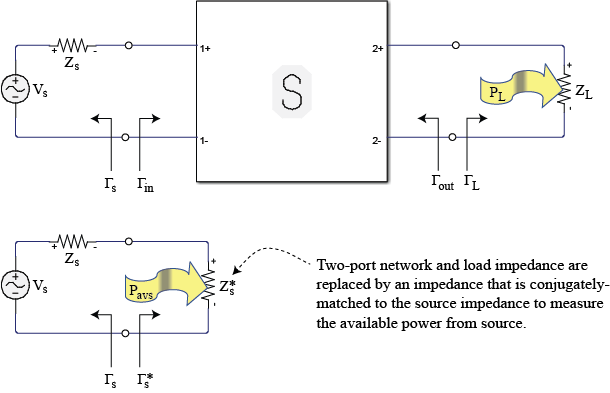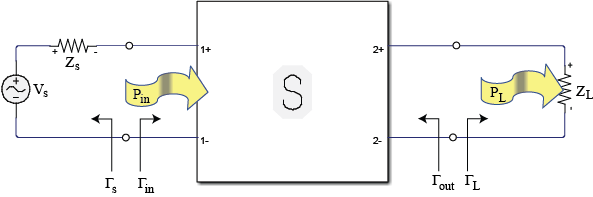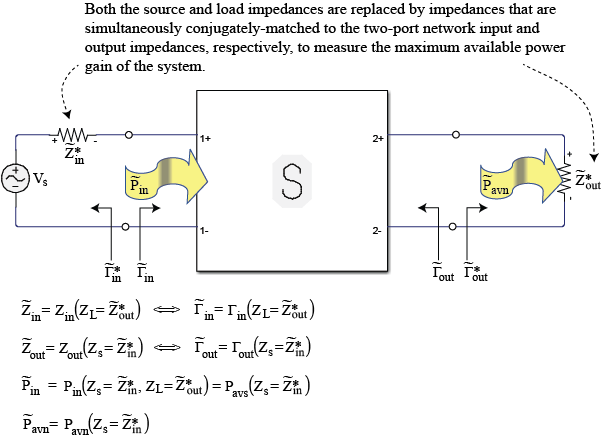powergain

Calculate power gain from two-port S-parameters

Description

g = powergain(s_params,z0,zs,zl,'Gt') calculates the transducer power gain of the 2-port network by:

${G}_{t}=\frac{{P}_{L}}{{P}_{\text{avs}}}=\frac{\left(1-{|{\Gamma }_{S}|}^{2}\right){|{S}_{21}|}^{2}\left(1-{|{\Gamma }_{L}|}^{2}\right)}{{|\left(1-{S}_{11}{\Gamma }_{S}\right)\left(1-{S}_{22}{\Gamma }_{L}\right)-{S}_{12}{S}_{21}{\Gamma }_{S}{\Gamma }_{L}|}^{2}}$

where,

• PL is the output power and Pavs is the maximum input power.

• ΓL and ΓS are the reflection coefficients defined as:

$\begin{array}{l}{\Gamma }_{S}=\frac{{Z}_{S}-{Z}_{0}}{{Z}_{S}+{Z}_{0}}\\ {\Gamma }_{L}=\frac{{Z}_{L}-{Z}_{0}}{{Z}_{L}+{Z}_{0}}\end{array}$

g = powergain(s_params,z0,zs,'Ga') calculates the available power gain of the 2-port network by:

${G}_{a}=\frac{{P}_{\text{avn}}}{{P}_{\text{avs}}}=\frac{\left(1-{|{\Gamma }_{S}|}^{2}\right){|{S}_{21}|}^{2}}{{|1-{S}_{11}{\Gamma }_{S}|}^{2}\left(1-{|{\Gamma }_{out}|}^{2}\right)}$

where

• Pavn is the available output power from the network.

• Γout is given by:

${\Gamma }_{\text{out}}={S}_{22}+\frac{{S}_{12}{S}_{21}{\Gamma }_{S}}{1-{S}_{11}{\Gamma }_{S}}$

g = powergain(s_params,z0,zl,'Gp') calculates the operating power gain of the 2-port network by:

${G}_{p}=\frac{{P}_{L}}{{P}_{\text{in}}}=\frac{{|{S}_{21}|}^{2}\left(1-{|{\Gamma }_{L}|}^{2}\right)}{\left(1-{|{\Gamma }_{\text{in}}|}^{2}\right){|1-{S}_{22}{\Gamma }_{L}|}^{2}}$

where

• Pin is the input power.

• Γin is given by:

${\Gamma }_{\text{in}}={S}_{11}+\frac{{S}_{12}{S}_{21}{\Gamma }_{L}}{1-{S}_{22}{\Gamma }_{L}}$

g = powergain(s_params,'Gmag') calculates the maximum available power gain of the 2-port network. by:

${G}_{\text{mag}}=\frac{|{S}_{21}|}{|{S}_{12}|}\left(K-\sqrt{{K}^{2}-1}\right)$

where K is the stability factor.

example

g = powergain(s_params,'Gmsg') calculates the maximum stable gain of the 2-port network by:

${G}_{msg}=\frac{|{S}_{21}|}{|{S}_{12}|}$

g = powergain(hs,zs,zl,'Gt') calculates the transducer power gain of the network represented by the S-parameter object hs.

g = powergain(hs,zs,'Ga') calculates the available power gain of the network represented by the S-parameter object hs.

g = powergain(hs,zl,'Gp') calculates the operating power gain of the network represented by the S-parameter object hs.

g = powergain(hs,'Gmag') calculates the maximum available power gain of the network represented by the S-parameter object hs.

g = powergain(hs,'Gmsg') calculates the maximum stable gain of the network represented by the S-parameter object hs.

Examples

collapse all

Calculate power gains for a sample 2-port network.

s11 = 0.61*exp(1j*165/180*pi);
s21 = 3.72*exp(1j*59/180*pi);
s12 = 0.05*exp(1j*42/180*pi);
s22 = 0.45*exp(1j*(-48/180)*pi);
sparam = [s11 s12; s21 s22];
z0 = 50;
zs = 10 + 1j*20;
zl = 30 - 1j*40;

Calculate the transducer power gain of the network

Gt = powergain(sparam,z0,zs,zl,'Gt')
Gt = 4.7066

Calculate the available power gain of the network

Ga = powergain(sparam,z0,zs,'Ga')
Ga = 11.4361

Note that, as expected, the available power gain is larger than the transducer power gain, Gt. The two become identical when Gt is measured with a matched load impedance:

zl_matched = gamma2z(gammaout(sparam, z0, zs)', z0);
Gt_zl_matched = powergain(sparam, z0, zs, zl_matched, 'Gt')
Gt_zl_matched = 11.4361

Calculate the operating power gain of the network

Gp = powergain(sparam,z0,zl,'Gp')
Gp = 10.5098

Note that, as expected, the operating power gain is larger than the transducer power gain, Gt. The two become identical when Gt is measured with a matched source impedance:

zs_matched = gamma2z(gammain(sparam, z0, zl)', z0);
Gt_zs_matched = powergain(sparam, z0, zs_matched, zl, 'Gt')
Gt_zs_matched = 10.5098

Calculate the maximum available power gain of the network

Gmag = powergain(sparam,'Gmag')
Gmag = 41.5032

Note that, as expected, the maximum available power gain is larger than the available power gain Ga, the transducer power gain, Gt, and the operating power gain, Gp. They all become identical when measured with simultaneously matched source and load impedances:

zs_matched_sim = gamma2z(gammams(sparam), z0);
zl_matched_sim = gamma2z(gammaout(sparam, z0, zs_matched_sim)', z0)
zl_matched_sim = 33.6758 + 91.4816i

That impedance can be also obtained directly using:

zl_matched_sim = gamma2z(gammaml(sparam), z0)
zl_matched_sim = 33.6758 + 91.4816i
Ga_matched_sim = powergain(sparam, z0, zs_matched_sim, 'Ga')
Ga_matched_sim = 41.5032
Gt_matched_sim = powergain(sparam, z0, zs_matched_sim, zl_matched_sim, 'Gt')
Gt_matched_sim = 41.5032
Gp_matched_sim = powergain(sparam, z0, zl_matched_sim, 'Gp')
Gp_matched_sim = 41.5032

When the scattering parameters represent a network that is not unconditionally stable, there is no set of source and load impedances that provide simultaneous matching. In this case, the maximum available power is infinite, but truly meaningless because the network is unstable.

To make the previously defined network conditionally stable, it is enough to increase the magnitude of the backward propagation scattering parameter, s12:

s12_cond_stable = 0.06*exp(1j*42/180*pi);
sparam_cond_stable = [s11 s12_cond_stable; s21 s22];

To verify that the network is conditionally stable, check that the stability factor, K, is smaller than 1:

K = stabilityk(sparam_cond_stable)
K = 0.9695

An attempt to calculate the maximum available gain of the network yields a NaN:

Gmag_cond_stable = powergain(sparam_cond_stable,'Gmag')
Gmag_cond_stable = NaN

Instead, the maximum stable gain, ${\mathit{G}}_{\mathrm{msg}}$, should be used.

Calculate the maximum stable power gain of the network

Gmsg_cond_stable = powergain(sparam_cond_stable,'Gmsg')
Gmsg_cond_stable = 62.0000

The maximum stable power gain is only meaningful when the network is not unconditionally stable.

Input Arguments

collapse all

2-port S-parameters, specified as an RF Toolbox™ S-parameter object.

2-port S-parameters, specified as a complex 2-by-2-by-N array.

Reference impedance in ohms, specified as a positive scalar. If the first input argument is an S-parameter object hs, the function uses hs.Impedance for the reference impedance.

Load impedance in ohms, specified as a positive scalar.

Source impedance in ohms, specified as a positive scalar.

Output Arguments

collapse all

Unit less power gain values, returned as a vector. To obtain power gain in decibels, use 10*log10(g).

If the specified type of power gain is undefined for one or more of the specified S-parameter values in s_params, the powergain function returns NaN. As a result, g is either NaN or a vector that contains one or more NaN entries.

collapse all

Transducer Power Gain

Gt = PL/Pavs is the ratio of power delivered to the load to the power available from the source. This depends on both ZS and ZL.Available Power Gain

Ga = Pavn/Pavs is the ratio of the power available from the two-port network to the power available from the source. Available gain is the transducer power gain when load impedance is equal to output impedance. Thus Ga depends only on Zs.Operating Power Gain

Gp = PL/Pin is the ratio of power dissipated in the load ZL to the power delivered to the input of the two-port network. This gain is independent of ZS, although some active circuits are strongly dependent on the input matching conditions.Maximum Available Power Gain and Maximum Stable Power Gain

Maximum available power gain, Gmag is Gawith matched input that is ZS is equal to Zin

In the case of conditionally stable two-port networks (K<1) where the maximum available power gain result is meaningless, the maximum stable power gain, Gmsg, should be used.